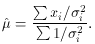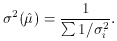4.5 The Weighted Mean

We have thus far discussed the estimation of the mean and standard deviation from a series of measurements of the same quantity with the same instrument. It often occurs, however, that one must combine two or more measurements of the same quantity with differing errors. A simple minded procedure would be to take the average of the measurements. This, unfortunately, ignores the fact that some measurements are more precise than others and should therefore be given more importance. A more valid method would be to weight each measurement in proportion to its error. The maximum likelihood method allows us to determine the weighting function to use.

From a statistics point of view, we have a sample x1, x1, . . , xn, where each value is from a Gaussian distribution having the same mean µ but a different standard deviationi. The likelihood function is thus the same as (45), but withreplaced byi. Maximizing this we then find the weighted mean(55)

Thus the weighting factor is the inverse square of the error, i.e., 1 /i2. This corresponds to our logic as the smaller thei, the larger the weight and vice-versa.

Using (33), the error on the weighted mean can now be shown to be(56)

Note that if all thei are the same, the weighted mean reduces to the normal formula in (49) and the error on the mean to (50).